## Arithmetic Operations

Note

These sections will help you understand what data type and scaling choices result in overflows or a loss of precision.

### Modulo Arithmetic

Binary math is based on modulo arithmetic. Modulo arithmetic uses only a finite set of numbers, wrapping the results of any calculations that fall outside the given set back into the set.

For example, the common everyday clock uses modulo 12 arithmetic. Numbers in this system can only be 1 through 12. Therefore, in the “clock” system, 9 plus 9 equals 6. This can be more easily visualized as a number circle: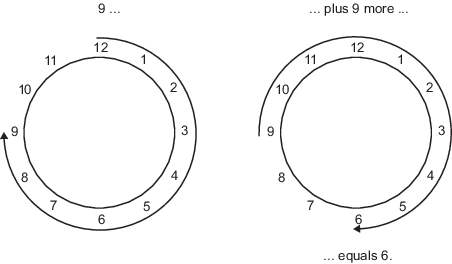Similarly, binary math can only use the numbers 0 and 1, and any arithmetic results that fall outside this range are wrapped “around the circle” to either 0 or 1.

### Two's Complement

Two's complement is a way to interpret a binary number. In two's complement, positive numbers always start with a 0 and negative numbers always start with a 1. If the leading bit of a two's complement number is 0, the value is obtained by calculating the standard binary value of the number. If the leading bit of a two's complement number is 1, the value is obtained by assuming that the leftmost bit is negative, and then calculating the binary value of the number. For example,

`$\begin{array}{l}01=\left(0+{2}^{0}\right)=1\\ 11=\left(\left(-{2}^{1}\right)+\left({2}^{0}\right)\right)=\left(-2+1\right)=-1\end{array}$`

To compute the negative of a binary number using two's complement,

1. Take the one's complement, or “flip the bits.”

2. Add a 2^(-FL) using binary math, where FL is the fraction length.

3. Discard any bits carried beyond the original word length.

For example, consider taking the negative of 11010 (-6). First, take the one's complement of the number, or flip the bits:

`$11010\to 00101$`

Next, add a 1, wrapping all numbers to 0 or 1:

`$\begin{array}{l}\underset{¯}{\begin{array}{r}00101\\ +1\end{array}}\\ \begin{array}{cc}00110& \left(6\right)\end{array}\end{array}$`

The addition of fixed-point numbers requires that the binary points of the addends be aligned. The addition is then performed using binary arithmetic so that no number other than 0 or 1 is used.

For example, consider the addition of 010010.1 (18.5) with 0110.110 (6.75):

`$\begin{array}{cc}\begin{array}{r}\underset{¯}{\begin{array}{c}010010.1\\ +0110.110\end{array}}\\ 011001.010\end{array}& \begin{array}{l}\left(18.5\right)\hfill \\ \left(6.75\right)\hfill \\ \left(25.25\right)\hfill \end{array}\end{array}$`

Fixed-point subtraction is equivalent to adding while using the two's complement value for any negative values. In subtraction, the addends must be sign-extended to match each other's length. For example, consider subtracting 0110.110 (6.75) from 010010.1 (18.5):

`$\begin{array}{cc}\underset{¯}{\begin{array}{c}010010.100\\ -0110.110\end{array}}& \begin{array}{c}\left(18.5\right)\\ \left(6.75\right)\end{array}\end{array}$`

The default fimath has a value of `1` (true) for the `CastBeforeSum` property. This casts addends to the sum data type before addition. Therefore, no further shifting is necessary during the addition to line up the binary points.

If `CastBeforeSum` has a value of `0` (false), the addends are added with full precision maintained. After the addition the sum is then quantized.

### Multiplication

The multiplication of two's complement fixed-point numbers is directly analogous to regular decimal multiplication, with the exception that the intermediate results must be sign-extended so that their left sides align before you add them together.

For example, consider the multiplication of 10.11 (-1.25) with 011 (3):

`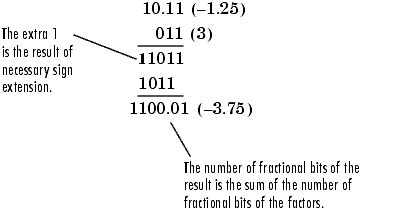`

#### Multiplication Data Types

The following diagrams show the data types used for fixed-point multiplication using Fixed-Point Designer™ software. The diagrams illustrate the differences between the data types used for real-real, complex-real, and complex-complex multiplication.

Real-Real Multiplication.  The following diagram shows the data types used by the toolbox in the multiplication of two real numbers. The software returns the output of this operation in the product data type, which is governed by the `fimath` object `ProductMode` property.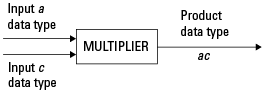Real-Complex Multiplication.  The following diagram shows the data types used by the toolbox in the multiplication of a real and a complex fixed-point number. Real-complex and complex-real multiplication are equivalent. The software returns the output of this operation in the product data type, which is governed by the `fimath` object `ProductMode` property: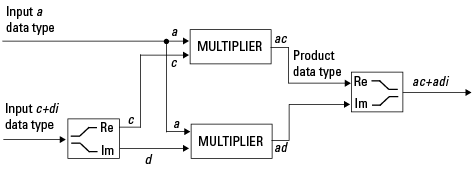Complex-Complex Multiplication.  The following diagram shows the multiplication of two complex fixed-point numbers. The software returns the output of this operation in the sum data type, which is governed by the `fimath` object `SumMode` property. The intermediate product data type is determined by the `fimath` object `ProductMode` property.When the `fimath` object `CastBeforeSum` property is `true`, the casts to the sum data type are present after the multipliers in the preceding diagram. In C code, this is equivalent to

```acc=ac; acc-=bd;```

for the subtractor, and

```acc=ad; acc+=bc;```

for the adder, where acc is the accumulator. When the `CastBeforeSum` property is `false`, the casts are not present, and the data remains in the product data type before the subtraction and addition operations.

#### Multiplication with fimath

In the following examples, let

```F = fimath('ProductMode','FullPrecision',... 'SumMode','FullPrecision'); T1 = numerictype('WordLength',24,'FractionLength',20); T2 = numerictype('WordLength',16,'FractionLength',10);```

Real*Real.  Notice that the word length and fraction length of the result `z` are equal to the sum of the word lengths and fraction lengths, respectively, of the multiplicands. This is because the `fimath` `SumMode` and `ProductMode` properties are set to `FullPrecision`:

```P = fipref; P.FimathDisplay = 'none'; x = fi(5, T1, F) x = 5 DataTypeMode: Fixed-point: binary point scaling Signedness: Signed WordLength: 24 FractionLength: 20 y = fi(10, T2, F) y = 10 DataTypeMode: Fixed-point: binary point scaling Signedness: Signed WordLength: 16 FractionLength: 10 z = x*y z = 50 DataTypeMode: Fixed-point: binary point scaling Signedness: Signed WordLength: 40 FractionLength: 30 ```

Real*Complex.  Notice that the word length and fraction length of the result `z` are equal to the sum of the word lengths and fraction lengths, respectively, of the multiplicands. This is because the `fimath` `SumMode` and `ProductMode` properties are set to `FullPrecision`:

```x = fi(5,T1,F) x = 5 DataTypeMode: Fixed-point: binary point scaling Signedness: Signed WordLength: 24 FractionLength: 20 y = fi(10+2i,T2,F) y = 10.0000 + 2.0000i DataTypeMode: Fixed-point: binary point scaling Signedness: Signed WordLength: 16 FractionLength: 10 z = x*y z = 50.0000 +10.0000i DataTypeMode: Fixed-point: binary point scaling Signedness: Signed WordLength: 40 FractionLength: 30 ```

Complex*Complex.  Complex-complex multiplication involves an addition as well as multiplication. As a result, the word length of the full-precision result has one more bit than the sum of the word lengths of the multiplicands:

```x = fi(5+6i,T1,F) x = 5.0000 + 6.0000i DataTypeMode: Fixed-point: binary point scaling Signedness: Signed WordLength: 24 FractionLength: 20 y = fi(10+2i,T2,F) y = 10.0000 + 2.0000i DataTypeMode: Fixed-point: binary point scaling Signedness: Signed WordLength: 16 FractionLength: 10 z = x*y z = 38.0000 +70.0000i DataTypeMode: Fixed-point: binary point scaling Signedness: Signed WordLength: 41 FractionLength: 30 ```

### Casts

The `fimath` object allows you to specify the data type and scaling of intermediate sums and products with the `SumMode` and `ProductMode` properties. It is important to keep in mind the ramifications of each cast when you set the `SumMode` and `ProductMode` properties. Depending upon the data types you select, overflow and/or rounding might occur. The following two examples demonstrate cases where overflow and rounding can occur.

Note

For more examples of casting, see Cast fi Objects.

#### Casting from a Shorter Data Type to a Longer Data Type

Consider the cast of a nonzero number, represented by a 4-bit data type with two fractional bits, to an 8-bit data type with seven fractional bits:As the diagram shows, the source bits are shifted up so that the binary point matches the destination binary point position. The highest source bit does not fit, so overflow might occur and the result can saturate or wrap. The empty bits at the low end of the destination data type are padded with either 0's or 1's:

• If overflow does not occur, the empty bits are padded with 0's.

• If wrapping occurs, the empty bits are padded with 0's.

• If saturation occurs,

• The empty bits of a positive number are padded with 1's.

• The empty bits of a negative number are padded with 0's.

You can see that even with a cast from a shorter data type to a longer data type, overflow can still occur. This can happen when the integer length of the source data type (in this case two) is longer than the integer length of the destination data type (in this case one). Similarly, rounding might be necessary even when casting from a shorter data type to a longer data type, if the destination data type and scaling has fewer fractional bits than the source.

#### Casting from a Longer Data Type to a Shorter Data Type

Consider the cast of a nonzero number, represented by an 8-bit data type with seven fractional bits, to a 4-bit data type with two fractional bits: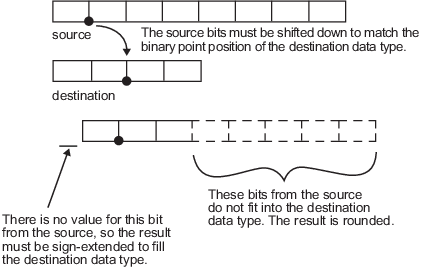As the diagram shows, the source bits are shifted down so that the binary point matches the destination binary point position. There is no value for the highest bit from the source, so sign extension is used to fill the integer portion of the destination data type. Sign extension is the addition of bits that have the value of the most significant bits to the high end of a two's complement number. Sign extension does not change the value of the binary number. In this example, the bottom five bits of the source do not fit into the fraction length of the destination. Therefore, precision can be lost as the result is rounded.

In this case, even though the cast is from a longer data type to a shorter data type, all the integer bits are maintained. Conversely, full precision can be maintained even if you cast to a shorter data type, as long as the fraction length of the destination data type is the same length or longer than the fraction length of the source data type. In that case, however, bits are lost from the high end of the result and overflow can occur.

The worst case occurs when both the integer length and the fraction length of the destination data type are shorter than those of the source data type and scaling. In that case, both overflow and a loss of precision can occur.

## SupportGet trial now